# MA.912.AR.5.3Export Print My Notes
Given a mathematical or real-world context, classify an exponential function as representing growth or decay.

### Clarifications

Clarification 1: Within the Algebra 1 course, exponential functions are limited to the forms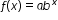, where b is a whole number greater than 1 or a unit fraction, or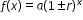, where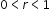.
General Information
Subject Area: Mathematics (B.E.S.T.)
Strand: Algebraic Reasoning
Status: State Board Approved

## Benchmark Instructional Guide

### Terms from the K-12 Glossary

• Exponential Function

### Vertical Alignment

Previous Benchmarks

Next Benchmarks

### Purpose and Instructional Strategies

In middle grades, students solved problems involving percentages, including percent increases and decreases. In Algebra I, students identify and describe exponential functions in terms of growth or decay rates. In later courses, students will further develop their understanding of exponential functions and how they are characterized by having a constant percent of change per unit interval.
• Provide opportunities to reference MA.912.AR.1.1 as students identify and interpret parts of an exponential equation or expression as growth or decay.
• Instruction includes the connection to growth or decay of a function as a key feature (constant percent rate of change) of an exponential function and being useful in understanding the relationships between two quantities.
• Instruction includes the use of graphing technology to explore exponential functions.
• For example, students can explore the function $f$($x$) =  $a$$b$$x$ and how the $a$-value and $b$-value are affected. Ask questions like “What impact does changing the value of $a$ have on the graph? What about $b$? What values for $b$ cause the function to increase? Which values cause it to decrease?” As students explore, formalize the terms exponential growth and decay when appropriate.
• As students explore the graph, have students choose values of $a$ and $b$ to complete a table of values. Once completed ask students what causes the value of $y$ to increase or decrease as the value of $x$ increases. Guide students to see that it’s because $b$ > 1 or $b$ < 1. Have students adjust the graph and repeat this exercise.
• Once students have an understanding of what causes exponential growth and decay, both graphically and algebraically, ask students if they think the curve ever passes $y$ = 0. Have students extend their function table for exponential decay to include more extreme values for $x$ to explore if it ever does. As student arrive at an understanding that it does not cross $y$ = 0, guide them to understand why it doesn’t algebraically. Once students arrive at this understanding, define this boundary as an asymptote.
• As students explore the provided graph, they will move the slider for $b$ to have negative values. The resulting graphs provide an interesting discussion point. Have students complete a function table using negative $b$ values. Students should quickly see the connection between the two “curves” and why neither is continuous. Let students know that for this reason, most contexts for exponential functions restrict $b$ to be greater than 0 and not equal to 1.
• As students solidify their understanding of $f$($x$) = $a$$b$$x$, use graphing technology again to have them explore the form $f$($x$) = $x$(1 ± $r$)$r$  . Guide students to use the sliders for $a$ and $r$ to visualize that only $r$ determines whether the function represents exponential growth or exponential decay.
• Have students discuss which values for $r$ cause exponential growth or decay. They should observe that negative values cause exponential decay while positive values cause exponential growth.

### Common Misconceptions or Errors

• Students may not understand exponential function values will eventually get larger than those of any other polynomial functions because they do not fully understand the impact of exponents on a value.
• Students may not understand that growth factors have one constraint ($b$ > 1) while decay factors have a compound constraint (0 < $b$ < 1). Some students may think that as long as $b$ < 1, the function will represent exponential decay.
• Students may think that if $a$ is negative and $r$ > 0 or $b$ > 1, the function represents an exponential decay. To address this misconception, help students understand that the negative values are growing at an exponential rate.

### Strategies to Support Tiered Instruction

• Teacher provides instruction to identify exponential functions in all methods (i.e., graphs, equations and tables).
• For example, instruction may include providing a comparison of the two forms of exponential functions. Having a side-by-side comparison of both as an equation, graph and a table of values will provide a visual aid.
• Teacher provides student with examples and non-examples of exponential functions in tables.
• For example, teacher can provide the following tables for students to determine which ones represent an exponential function.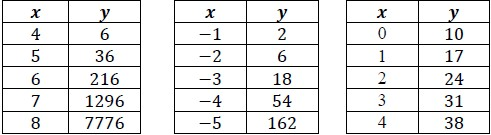• After a person takes medicine, the amount of drug left in the person’s body changes over time. When testing a new drug, a pharmaceutical company develops a mathematical model to quantify this relationship. To find such a model, suppose a dose of 1000 mg of a certain drug is absorbed by a person’s bloodstream. Blood samples are taken every five hours, and the amount of drug remaining in the body is calculated. The data collected from a particular sample is recorded below.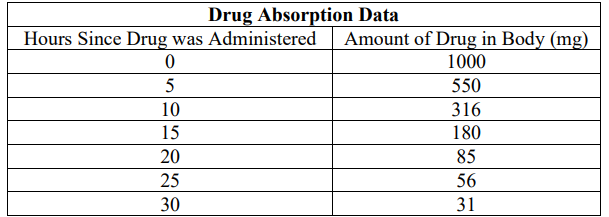• Part A. Does this data represent an exponential growth or decay function? Justify your answer.
• Part B. Create an exponential function that describes the data in the table above.

### Instructional Items

Instructional Item 1
• Given the function $f$($x$) = 125(1 − 0.26), does it represent an exponential growth or decay function?

*The strategies, tasks and items included in the B1G-M are examples and should not be considered comprehensive.

## Related Courses

This benchmark is part of these courses.
1200310: Algebra 1 (Specifically in versions: 2014 - 2015, 2015 - 2022, 2022 and beyond (current))
1200320: Algebra 1 Honors (Specifically in versions: 2014 - 2015, 2015 - 2022, 2022 and beyond (current))
1200380: Algebra 1-B (Specifically in versions: 2014 - 2015, 2015 - 2022, 2022 and beyond (current))
1200400: Foundational Skills in Mathematics 9-12 (Specifically in versions: 2014 - 2015, 2015 - 2022, 2022 and beyond (current))
7912070: Access Mathematics for Liberal Arts (Specifically in versions: 2014 - 2015, 2015 - 2018, 2018 - 2019, 2019 - 2022, 2022 - 2023, 2023 and beyond (current))
7912090: Access Algebra 1B (Specifically in versions: 2014 - 2015, 2015 - 2018, 2018 - 2019, 2019 - 2022, 2022 and beyond (current))
1200315: Algebra 1 for Credit Recovery (Specifically in versions: 2014 - 2015, 2015 - 2022, 2022 and beyond (current))
1200385: Algebra 1-B for Credit Recovery (Specifically in versions: 2014 - 2015, 2015 - 2022, 2022 and beyond (current))
7912075: Access Algebra 1 (Specifically in versions: 2014 - 2015, 2015 - 2018, 2018 - 2019, 2019 - 2022, 2022 and beyond (current))
1207350: Mathematics for College Liberal Arts (Specifically in versions: 2022 and beyond (current))
1700600: GEAR Up 1 (Specifically in versions: 2020 - 2022, 2022 and beyond (current))
1700610: GEAR Up 2 (Specifically in versions: 2020 - 2022, 2022 and beyond (current))
1700620: GEAR Up 3 (Specifically in versions: 2020 - 2022, 2022 and beyond (current))
1700630: GEAR Up 4 (Specifically in versions: 2020 - 2022, 2022 and beyond (current))

## Related Access Points

Alternate version of this benchmark for students with significant cognitive disabilities.
MA.912.AR.5.AP.3: Given a real-world context, identify an exponential function as representing growth or decay.

## Related Resources

Vetted resources educators can use to teach the concepts and skills in this benchmark.

## Formative Assessments

Trees in Trouble:

Students are asked to write a function that represents an annual loss of 3 percent each year.

Type: Formative Assessment

Exponential Growth:

Students are given two functions, one represented verbally and the other by a table, and are asked to compare the rate of change in each in the context of the problem.

Type: Formative Assessment

Exponential Functions - 2:

Students are asked to identify the percent rate of change of a given exponential function.

Type: Formative Assessment

Exponential Functions - 1:

Students are asked to identify the percent rate of change of a given exponential function.

Type: Formative Assessment

## Original Student Tutorials

Exponential Functions Part 3: Decay:

Learn about exponential decay as you calculate the value of used cars by examining equations, graphs, and tables in this interactive tutorial.

Type: Original Student Tutorial

Exponential Functions Part 2: Growth:

Learn about exponential growth in the context of interest earned as money is put in a savings account by examining equations, graphs, and tables in this interactive tutorial.

Type: Original Student Tutorial

Exponential Functions Part 1:

Learn about exponential functions and how they are different from linear functions by examining real world situations, their graphs and their tables in this interactive tutorial.

Type: Original Student Tutorial

## Perspectives Video: Professional/Enthusiast

Making Candy: Illuminating Exponential Growth:

No need to sugar coat it: making candy involves math and muscles. Learn how light refraction and exponential growth help make candy colors just right!

Type: Perspectives Video: Professional/Enthusiast

## MFAS Formative Assessments

Exponential Functions - 1:

Students are asked to identify the percent rate of change of a given exponential function.

Exponential Functions - 2:

Students are asked to identify the percent rate of change of a given exponential function.

Exponential Growth:

Students are given two functions, one represented verbally and the other by a table, and are asked to compare the rate of change in each in the context of the problem.

Trees in Trouble:

Students are asked to write a function that represents an annual loss of 3 percent each year.

## Original Student Tutorials Mathematics - Grades 9-12

Exponential Functions Part 1:

Learn about exponential functions and how they are different from linear functions by examining real world situations, their graphs and their tables in this interactive tutorial.

Exponential Functions Part 2: Growth:

Learn about exponential growth in the context of interest earned as money is put in a savings account by examining equations, graphs, and tables in this interactive tutorial.

Exponential Functions Part 3: Decay:

Learn about exponential decay as you calculate the value of used cars by examining equations, graphs, and tables in this interactive tutorial.

## Student Resources

Vetted resources students can use to learn the concepts and skills in this benchmark.

## Original Student Tutorials

Exponential Functions Part 3: Decay:

Learn about exponential decay as you calculate the value of used cars by examining equations, graphs, and tables in this interactive tutorial.

Type: Original Student Tutorial

Exponential Functions Part 2: Growth:

Learn about exponential growth in the context of interest earned as money is put in a savings account by examining equations, graphs, and tables in this interactive tutorial.

Type: Original Student Tutorial

Exponential Functions Part 1:

Learn about exponential functions and how they are different from linear functions by examining real world situations, their graphs and their tables in this interactive tutorial.

Type: Original Student Tutorial

## Perspectives Video: Professional/Enthusiast

Making Candy: Illuminating Exponential Growth:

No need to sugar coat it: making candy involves math and muscles. Learn how light refraction and exponential growth help make candy colors just right!

Type: Perspectives Video: Professional/Enthusiast

## Parent Resources

Vetted resources caregivers can use to help students learn the concepts and skills in this benchmark.

## Perspectives Video: Professional/Enthusiast

Making Candy: Illuminating Exponential Growth:

No need to sugar coat it: making candy involves math and muscles. Learn how light refraction and exponential growth help make candy colors just right!

Type: Perspectives Video: Professional/Enthusiast